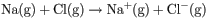# Problem: The following reaction can be written as the sum of two reactions, one of which relates to ionization energy and one of which relates to electron affinity:a. What is the reaction that corresponds to the first ionization energy of sodium, Na?b. What is the reaction that corresponds to the electron affinity of chlorine, Cl?

###### FREE Expert Solution

Ionization energy is defined as the minimum energy required to remove a single valence electron from an isolated gaseous atom.

Electron affinity is defined as the amount of energy released or absorbed when a single electron is added to the valence shell of an isolated gaseous atom.

79% (186 ratings)###### Problem Details

The following reaction can be written as the sum of two reactions, one of which relates to ionization energy and one of which relates to electron affinity:a. What is the reaction that corresponds to the first ionization energy of sodium, Na?

b. What is the reaction that corresponds to the electron affinity of chlorine, Cl?